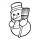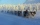# Thermometer

thermometer showed -12 degrees Celsius at the morning then the temperature rises by 4 degrees and later again increased by 2 degrees at the evening has fallen by 5 degrees and then fall 3 degrees. What end temperature does the thermometer show?

Result

t =  -14 °C

#### Solution:Leave us a comment of example and its solution (i.e. if it is still somewhat unclear...):

Showing 0 comments:Be the first to comment!## Next similar examples:

1. ThermometerThe thermometer showed -3°C at morning. Then temperature was increased by 1°C again increased by 1°C and then decreased by 1°C and then decreased by 4°C. Which terminal temperature thermometer shows?
2. This morningThis morning it was 80 degrees F outside the temperature increased 12 degrees F. What is the temperature now?
3. Temperature 2Sunday's high temperature was 3 degrees higher than Saturday's. On Monday, the temperature fell 5 degrees, then rose 7 degrees on Tuesday and 4 more on Wednesday. Then it fell 17 degrees to a record low of 31 on Thursday. What was the temperature on Saturd
4. The temperartureThe temperarture is 0 degrees. It drops 5 degrees after 1 hour and then drops 3 degrees more the next hour. What is the temperature after 6 hours?
5. In aboutIn about 12 hours in North Dakota the temperature rose from -33 degrees farenheit to 50 degrees farenheit. By how much did the temperature change?
6. Temperature addingAt a weather centre, the temperature at midnight was -2 degree Celsius and by noon it had raised 4 degree Celsius. What is the new temperature?
7. NoontimeIn the morning temperature was 110°F. By noontime it has gone up by 15°F. What was the noon temperature. (Give your answer in °F)
8. Roses and tulipsAt the florist are 50 tulips and 5 times less roses. How many flowers are in flower shop?
9. Change in temperatureStarting temperature is 21°C, the highest temperature is 32°C. What is the change in temperature?
10. The temperatureThe temperature at 1:00 was 10 F. Between 1:00 and 2:00, the temperature dropped 15F. Between 2:00 and 3:00, the temperature rose 3F. What is the temperature at 3:00?
11. Roman numerals 2+Add up the number writtens in Roman numerals. Write the results as a roman numbers.
12. Roman numerals +Add up the number writtens in Roman numerals. Write the results as a decimal number.
13. Addition of Roman numbersAdded together and write as decimal number: LXVII + MLXIV
14. Adding mixed numbersAdd this two mixed numbers: 1 5/6 + 2 2/11=
15. Rounding 2Round the following negative number: -143.021
16. Evaluate expression 2Evaluate expression with negatives: (-3)+4+(-8)+(-6)+4+(-1)
17. Addition of mixed numeralsAdd two mixed fractions: 2 4/6 + 1 3/6